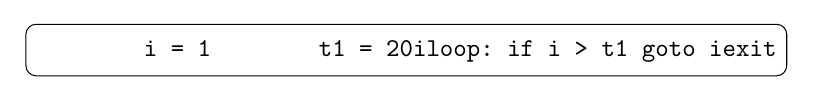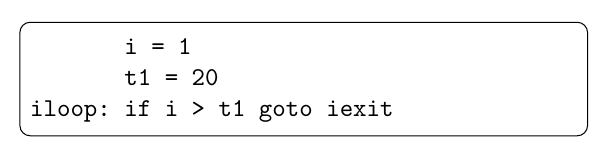typing environment inside tikz node with ConTeXt

I want to render some lines of code inside a tikz node:

\usemodule[tikz]
\definetyping[code][bodyfont=small,escape={`,`}]

\starttext
\tikzset{
code/.style={rectangle, rounded corners, draw=black}
}
\starttikzpicture[node distance=.7cm]
\node[code] (g1) {
\startcode
i = 1
t1 = 20
iloop: if i > t1 goto iexit
\stopcode
};
\stoptikzpicture
\stoptext

Which renders:As you can see, all line breaks are missing. I managed to enhance this by using paragraphs:

\usemodule[tikz]
\definetyping[code][bodyfont=small,escape={`,`}]

\defineparagraphs[CodeNode][n=1]
\setupparagraphs[CodeNode][width=.5\textwidth]

\starttext
\tikzset{
code/.style={rectangle, rounded corners, draw=black}
}
\starttikzpicture[node distance=.7cm]
\node[code] (g1) {
\startCodeNode
\startcode
i = 1
t1 = 20
iloop: if i > t1 goto iexit
\stopcode
\stopCodeNode
};
\stoptikzpicture
\stoptext

This renders:Which is more close to what I want, but I now needed to give a specific width. How can I create a node that automatically encloses the given code without giving a width?

Encapsulate the code in a framed environment. (Using »Making a tight vertical fit« from the Garden)

\usemodule[tikz]

\defineframed
[codeframed]
[frame=no,
width=fit,
align=right,
strut=no,
offset=0pt]

\definetyping
[code]
[bodyfont=small,
escape={`,`},
before={\startframed[codeframed]},
after={\stopframed}]

\starttext

\tikzset{
code/.style={rectangle, rounded corners, draw=black}
}
\starttikzpicture[node distance=.7cm]
\node[code] (g1) {
\startcode
i = 1
t1 = 20
iloop: if i > t1 goto iexit
\stopcode
};
\stoptikzpicture

\stoptextThe same can be achieved using framedtext which looks, in my opinion, a little bit cleaner than the framed solution. (Thanks to Hans for pointing out location=none)

\usemodule[tikz]

\defineframedtext
[codeframed]
[frame=no,
width=fit,
location=none,
offset=0pt]

\definetyping
[code]
[bodyfont=small,
escape={`,`},
before={\startcodeframed},
after={\stopcodeframed}]

\starttext

\tikzset{
code/.style={rectangle, rounded corners, draw=black}
}
\starttikzpicture[node distance=.7cm]
\node[code] (g1) {
\startcode
i = 1
t1 = 20
iloop: if i > t1 goto iexit
\stopcode
};
\stoptikzpicture

\stoptextIf the usage of TikZ is not compulsory, you might want to use framedtext alone.

\defineframedtext
[codeframed]
[autowidth=force,corner=round]

\definetyping
[code]
[bodyfont=small,
escape={`,`},
before={\startcodeframed},
after={\stopcodeframed}]

\starttext

\startcode
i = 1
t1 = 20
iloop: if i > t1 goto iexit
\stopcode

\stoptext